Courses

# Mechanical Properties Of Materials - 1

## 10 Questions MCQ Test Topicwise Question Bank for GATE Mechanical Engineering | Mechanical Properties Of Materials - 1

Description
This mock test of Mechanical Properties Of Materials - 1 for Mechanical Engineering helps you for every Mechanical Engineering entrance exam. This contains 10 Multiple Choice Questions for Mechanical Engineering Mechanical Properties Of Materials - 1 (mcq) to study with solutions a complete question bank. The solved questions answers in this Mechanical Properties Of Materials - 1 quiz give you a good mix of easy questions and tough questions. Mechanical Engineering students definitely take this Mechanical Properties Of Materials - 1 exercise for a better result in the exam. You can find other Mechanical Properties Of Materials - 1 extra questions, long questions & short questions for Mechanical Engineering on EduRev as well by searching above.
QUESTION: 1

Solution:
QUESTION: 2

Solution:
QUESTION: 3

### Young's modulus is defined as the ratio of

Solution:

The linear relationship between stress and strain for a bar in simple tension or compression can be expressed by the equation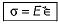in which Eis a constant of proportionality known as modulus of elasticity for the material. The modulus of elasticity is the slope of the stress- strain diagram in the linearly elastic region and its value depends upon the particular material being used.

QUESTION: 4

Match List-I (Material) with List-ll (Young’s modulus):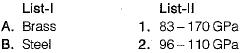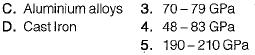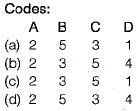Solution: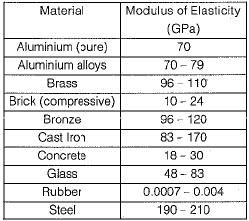QUESTION: 5

Match List-I with List-ll (Material):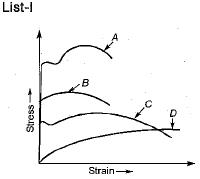List-ll
1. Cold rolled steel
2. Low carbon steel
3. Medium carbon steel heat treated
4. Soft brass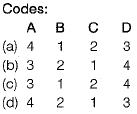Solution:
QUESTION: 6

A thin mid steel wire is loaded by adding loads in equal increments till it breaks. The extensions noted with increasing loads will behave as under

Solution:
QUESTION: 7

Match List-1 (Materia!) with List-ll (Poisson’s Ratio)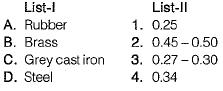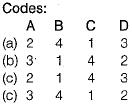Solution:
QUESTION: 8

During a tensile test on a specimen of 1 cm2 cross-section, maximum load observed was 80 kN and area of cross-section at neck was 0.5 cm2. UTS of specimen is

Solution: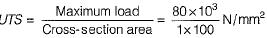= 800 MPa

QUESTION: 9

A prismatic bar of circular cross-section is loaded by tensile forces P= 90 kN. The bar has length L = 3 m and diameter d = 30 mm. If is made of aluminium with modulus of elasticity E = 70 GPa and Poisson’s ratio v = 1/3. What is the increase in volume ΔV of the bar?

Solution: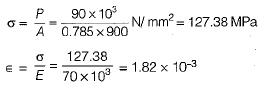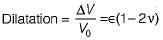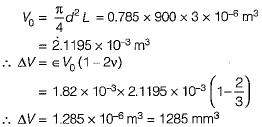QUESTION: 10

Resilience of a material is considered when it is subjected to

Solution:

Resilience represent the ability of the material to absorb energy within the elastic range.